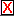# Sine Wave Geometry

In the illustration below, X is a point on the yellow circle, and time is a point on the horizontal segment (axis). Drag each of these points with the mouse.

The red line passing horizontally through X isolates the height of X above (or below) the axis. This "height" of a point on a unit circle is known as the sine of the angle determined by that point (from the center of the circle). Thus, the red line represents sin(X) geometrically. As you drag X around the circle, sin(X) rises and falls.

The blue line passing vertically through time simply represents a single moment of time. As you drag time along its axis, the given moment of time is "earlier" (to the left) or "later" (to the right).

The point P falls at the intersection of the red and blue lines. It, therefore, represents the sine of X (because it falls on the red line) at a given moment in time (because it falls on the blue line). As you drag things, this point leaves behind a trace of where it has been.

You can clear these traces whenever you want by clicking the.